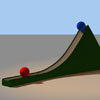#### You may also like### Whoosh

A ball whooshes down a slide and hits another ball which flies off the slide horizontally as a projectile. How far does it go?

# Dam Busters 2

### Why do this problem?

This problem links three fundamental parts of mechanics: dimensional analysis, kinematics and coefficients of restitution. Students will gain understanding of how equations are made. They will apply dimensional analysis in a meaningful context. The interesting situation will provide interest for the topic of kinematics. The context is sufficiently rich to allow extrapolation by the able student.

### Possible approach

Students could be given time to look at form of the possible equations. Although they might look complicated, the complexity is largely symbolic.

Then students could be encouraged to consider work out which equations might not work. They should be encouraged to give their reasons clearly. This could be done in pairs.

### Key questions

• What are the key features of the equations?
• What conditions must sensible physical equations satisfy?
• Are there any equations which will obviously not work?
• How would the analysis for a real bomb vary (discussion)?
• Do you think that effects of air resistance etc. will be significant (discussion)?

### Possible extension

Students can work on the derivation of the equation. For even more extension, they could try to analyse the 3 bounce case or even try to work out the effects of an infinite number of bounces.

### Possible support

Students struggling could try the first question Dam Busters 1.

If they are struggling on the dimensional analysis part then you could ask them to work out the dimensions of g and velocities directly.